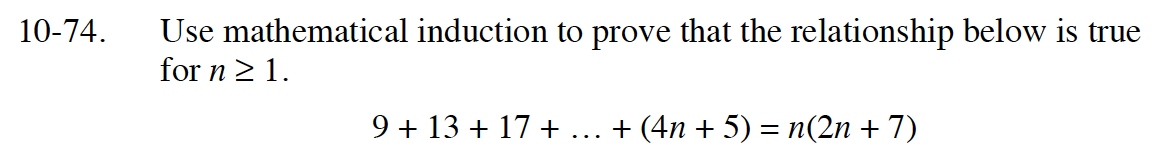### Home > INT3 > Chapter Ch10 > Lesson 10.1.5 > Problem10-74

10-74.
1. Use mathematical induction to prove that the relationship below is true for n ≥ 1. Homework Help ✎

2. 9 + 13 + 17 + … + (4n + 5) = n(2n + 7)Prove the relationship is true for n = 1.
9 = (1)(2(1) + 7)
9 = 9

Assume that the relationship is true for some number k.

Prove that, if the relationship is true for k, then it is true for k + 1.